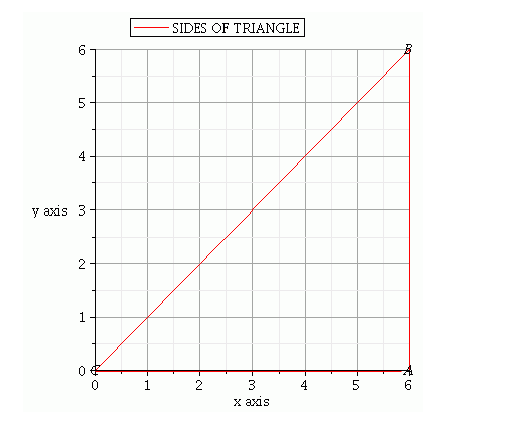# The area of the triangle formed by the lines y = x, x = 6 and y = 0 is`
Question:

The area of the triangle formed by the lines y = x= 6 and y = 0 is

(a) 36 sq. units

(b) 18 sq. units

(c) 9 sq. units

(d) 72 sq. units

Solution:

Given $x=6, y=0$ and $x=y$

We have plotting points as $(6,0)(0,0)(6,6)$ when $x=y$Therefore, area of $\triangle A B C=\frac{1}{2}($ Base $\times$ Height $)=\frac{1}{2}(C A \times A B)=\frac{1}{2}(6 \times 6)=\frac{1}{2} \times 36=18$

Area of triangle $A B C$ is 18 square units

Hence, the correct choice is $b$